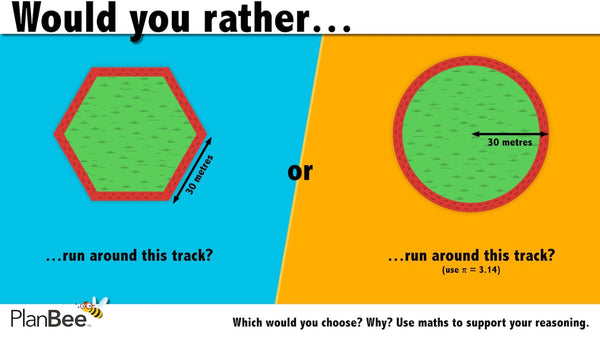# Would you rather…? Maths reasoning questions № 2

Here's another Maths reasoning question for KS1 or KS2 Maths lessons. It's ideal for displaying on your interactive whiteboard or display. It's the second in our new series of 'Would you rather…?' Maths reasoning questions.

These fun, visual problems are challenging, but open to interpretation by your pupils. For many of them, there is no 'right answer', but a choice of answers which children must first identify and choose from, then justify their choice using maths.

You'll find an explanation of the Maths involved in this 'Would you rather…?' Maths reasoning question directly underneath it, as well as links to all of the other questions in this problem-solving series.

## 'Would you rather…?' Maths reasoning question № 2:Would you rather run around the hexagonal track or the circular track?

### Teacher's Notes

You may wish to give children the following clue:

The formula for calculating circumference is 2πr (2 x pi x radius).

The 'solution':

The perimeter of the hexagonal track is:

6 x 30 = 180m

The perimeter/circumference of the circular track is:

2 x 3.14 x 30 = 188.4m

If your aim is to run the shortest distance, the hexagonal track is preferable.

Rounding pi to two decimal places makes it easier for children to use written multiplication methods to solve the problem. Alternatively, they could use calculators. They might have reasons for choosing either track but stress that whichever they choose, they justify their reason mathematically.

Check out the other 'Would you rather…?' Maths reasoning questions.

Looking for more Maths Problem Solving lessons and plans? Check out our ready-to-teach collection here.